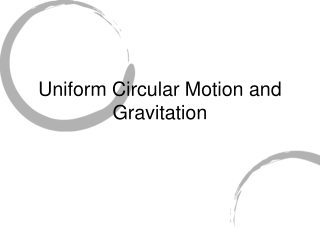DownloadDownload PresentationUniform Circular Motion and Gravitation

# Uniform Circular Motion and Gravitation

Download Presentation## Uniform Circular Motion and Gravitation

- - - - - - - - - - - - - - - - - - - - - - - - - - - E N D - - - - - - - - - - - - - - - - - - - - - - - - - - -
##### Presentation Transcript

1. Uniform Circular Motion and Gravitation

2. UCM • What is UCM? It describes an object that moves in a circle with a constant speed • Implications of UCM… An object accelerates without changing its speed. The direction continuously changes as an object moves around a circle

3. UCM • Newton’s first law says that an object in motion continues in motion with a constant velocity unless acted upon by a net external force. • Velocity is a vector: speed and direction. • A constant velocity means constant speed AND direction. • Direction changes, therefore a force was applied!

4. UCM • An object’s natural path is a straight line, therefore to pull an object out of its natural path a net force was applied • What is this force? This force could be any of our everyday forces (weight, normal, tension, applied, or friction) or a combination of those five. It is the force or forces that maintain(s) the circle • Does this force have a name? The Centripetal Force (center seeking), Fc

5. UCM • If there is a net force, is there an acceleration? Of course, ΣF = ma after all! • Does this acceleration have a name? The Centripetal Acceleration, ac

6. UCM • What about direction? The force and acceleration have the same direction, F and a, but what is it? Towards the CENTER of the circle

7. UCM • What is the equation for acceleration?

8. UCM • What is the equation for centripetal force? • Remember that Fc is the net force toward the center of the circle.

9. Examples of Fc

10. Examples of Fc

11. Examples of Fc

12. Examples of Fc

13. Examples of Fc

14. Centripetal vs Centrifugal • The centrifugal force is a fictional force. It is not real! It is usually used in place of the correct Newton’s First Law when explaining certain behaviors exhibited in circular motion. • Go forth and enlighten those that unknowingly spread incorrect physics.

15. Newton’s Law of Universal Gravitation • Every particle in the universe attracts every other particle in the universe with a force that is proportional to the product of the masses and inversely proportional to the square of the distances between them. The force acts along the line joining the two points.

16. Newton’s Law of Universal Gravitation

17. Gravity Strength on Earth

18. Satellites and “weightlessness” • Two types of weightlessness: • Apparent: free fall • Real: Away from all massive bodies • Satellites orbit because of the gravitational force

19. Johannes Kepler • 1571 – 1630 • Assistant to Tycho Brahe (1546 – 1601) from 1599 – 1601 • Replaced Copernicus’ circular orbits with ellipses (25 years of calculating to do this)

20. Cavendish